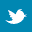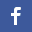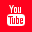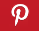Completely Solved C, C++ Programs Assignment.Quick Search Database Videos Tutorials Ebooks Forums FAQ Aboutus Household Industrial Manufacturing Service Shopping Transportation### C Program to print the pascal's triangle recursively.

 Filed Under: C Assignments

Program Statement:
Print the pascal's triangle recursively.

Theory:
In mathematics, Pascal's triangle is a geometric arrangement of the binomial coefficients in a triangle. The rows of Pascal's triangle are conventionally enumerated starting with row 0, and the numbers in each row are usually staggered relative to the numbers in the adjacent rows. A simple construction of the triangle proceeds in the following manner. On row 0, write only the number 1. Then, to construct the elements of following rows, add the number directly above and to the left with the number directly above and to the right to find the new value. If either the number to the right or left is not present, substitute a zero in its place. For example, the first number in the first row is 0 + 1 = 1, whereas the numbers 1 and 3 in the third row are added to produce the number 4 in the fourth row.

Algorithm:
Algo_pascal(int r,int c)
This function recursively calculates and returns the element (which is an integer) at rth row and cth column.
{
For matching the first column and last column entries.
if(c=0 OR r=c)
return 1;
else
return Algo_pascal(r-1,c-1)+Algo_pascal(r-1,c);
}
End of algorithm

Program listing:
/*C programme to print pascal's triangle recursively*/
#include<stdio.h>
main()
{
int i,j,k,h,p;
int pascal(int,int);                          /*Initialization of the function prototype*/
printf("Enter height of the triangle : ");
scanf("%d",&h);                  /*The height of the triangle is taaken as input by the user*/ for(i=0;i<h;i++)                        /*For loop used as row to calculate pascal's triangle*/
{
for(k=i;k<h;k++)                    /*For loop to print pascal's triangle in triangular form*/
printf(" ");
for(j=0;j<=i;j++)                      /*For loop used as column to calculate pascal's triangle*/
{
p = pascal(i,j);                              /*Function call*/
printf("%4d",p);                              /*"%4d" is used to print 4 blank spaces between two characters*/
}
printf("\n");
}
}
int pascal(int r,int c)                             /*Function definiition to calculate pascal's triangle*/
{
if(c==0 || r==c)
return 1;
else
return pascal(r-1,c-1)+pascal(r-1,c);                            /*Recursive call*/
}

Output :
Enter height of the triangle : 10

1
1   1
1   2   1
1   3   3   1
1   4   6   4   1
1   5  10  10   5   1
1   6  15  20  15   6   1
1   7  21  35  35  21   7   1
1   8  28  56  70  56  28   8   1
1   9  36  84 126 126  84  36   9   1

Discussions :
● Pascal's triangle can also be done non-recursively by using combination method, i.e. nCr where n is the number of rows(starting from 0) and r is the number of columns(starting from 0).
● Since pascal's triangle is done recursively therefore it requires a lot of memory space as the functions are stacked up in main memory until an end is reached.Back to main directory:Software Practical